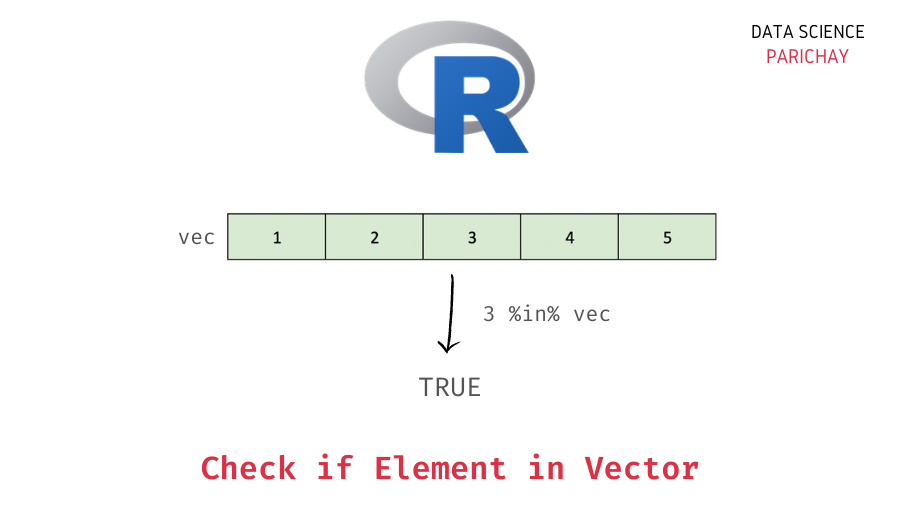# Check if an Element is present in an R Vector

Vectors are a commonly used data structure in R. They are used to store sequential (and one-dimensional) values of the same type. In this tutorial, we will look at how to check if an element is present in an R vector with the help of some examples.

## How to check if a vector contains an element in R?

You can use the `%in%` operator in R to check if an element is present in a vector or not. The following is the syntax –

```# check if element e is present in vector vec
e %in% vec```

It returns `TRUE` if the value is present in the vector and `FALSE` if it’s not.

## Examples

Let’s look at some examples of using the above syntax to check if a vector contains a given element or not. First, we will create a vector that we will be using throughout this tutorial.

```# create a vector
vec <- c(2, 4, 6, 8, 10)
# display the vector
print(vec)```

Output:

`  2  4  6  8 10`

We have a vector of even numbers from 2 to 10.

### Using `%in%` to check if an element is present in a vector

Let’s check if the element `4` is present in the above vector.

```# check if 4 is present in vec
4 %in% vec```

Output:

📚 Data Science Programs By Skill Level

Introductory

Intermediate ⭐⭐⭐

🔎 Find Data Science Programs 👨‍💻 111,889 already enrolled

Disclaimer: Data Science Parichay is reader supported. When you purchase a course through a link on this site, we may earn a small commission at no additional cost to you. Earned commissions help support this website and its team of writers.

`TRUE`

We get `TRUE` as the output since the element `4` is present in the vector, `vec`.

Let’s look at another example, this time we’ll check with a value that is not present in the above vector. For example, 5.

```# check if 5 is present in vec
5 %in% vec```

Output:

`FALSE`

We get `FALSE` as the output as the element `5` is not present in the above vector.

### Using `is.element()` to check if an element is present in a vector

Alternatively, you can also use the `is.element()` function in R to check if an element is present in a vector or not. For this, pass the element as the first argument and the vector as the second argument.

Let’s use the same example as above. We’ll check whether the element `4` is present in the vector `vec` or not.

```# check if 4 is present in vec
is.element(4, vec)```

Output:

`TRUE`

We get the same result as above.

You might also be interested in –

•